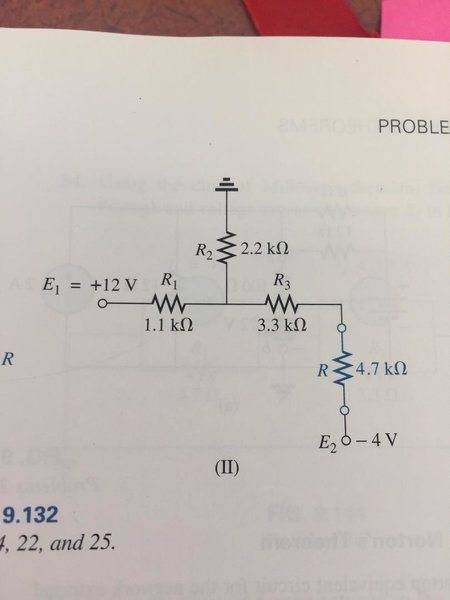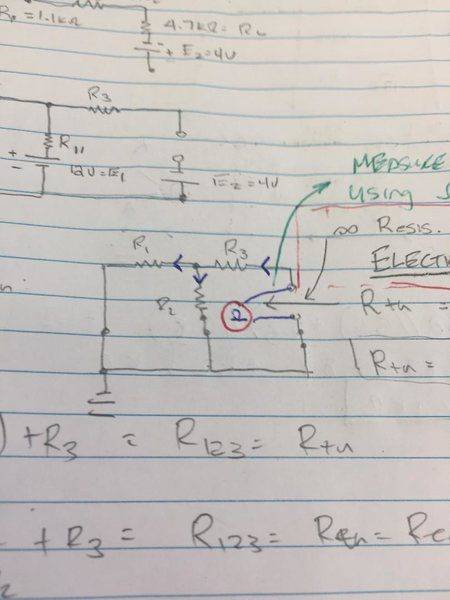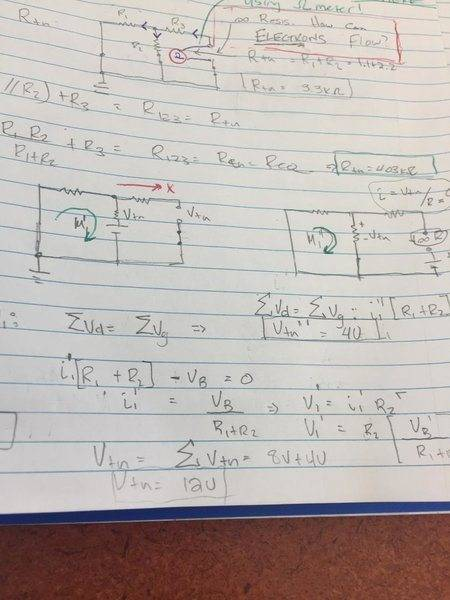# Thevenin equivalent circuit

• Engineering
• icesalmon

## Homework Statement

I just want to check to see if my understanding in anything here has gaps.

Q: Find the Thevenin equivalent circuit for the network external to he load resistor ##R_L##:## Homework Equations

Ohm's Law: ## V = IR ##
Kirchoff's Voltage Law: Σ##V_{drops}## + Σ##V_{gains}## = 0
Kirchoff's Current Law: Σ##I_{closed loop}## = 0

## The Attempt at a Solution

I started by redrawing the circuit as best as I could

For the thevenin resistance I removed ##R_L## with an open circuit and then short circuit the 12V source and place an ohm-meter at the open circuit where ##R_L## was removed:Calculating the thevenin resistance: I get ##R_{th} = ( R_1 // R_2 ) + R_3 = 4.04kΩ##

Placing the ##12V## source back in and calculating the thevenin voltage ##V_{th}## would be the voltage drop across the resistor ##R_2##.

Using superposition I can determine the effects each voltage source has on ##R_2##
starting by shorting out the ##4V## source I get the network on the leftSince there is an open circuit where ##R_L## was removed current will not flow through that branch and I can calculate ##I'## by using KVL:
Σ##V_{drops}## = Σ##V_{gains}##: ## -12V + I_1R1 + I_1R2 = 0 ## ##I_1## => ##I_1'R_2## => ##V_{th}' = 8V##

Now removing the 12V Source ##E_1## and seeing that ##E_2## is in parallel with ##R_2## I can automatically determine ##V_th##'' to be ##E_2 = 4V##

From superposition: ##V_{th} = V_{th}' + V_{th}''## ##V_th = 8V + 4V = 12V##

Correct.